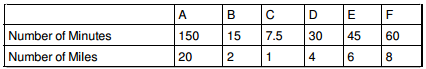• 10/31/13   |   Adjusted: 08/01/18   |   1 file# Riding at a Constant Speed, Assessment Variation

• Description
• Files

Mathematically:

• Addresses standards: 6.RP.A.2, 6.RP.A.3MP.2, and MP.6
• Provides a simply stated, yet mathematically rich task for standards 6.RP.A.2 and 6.RP.A.3
• Requires students to think about proportional relationships flexibly and with different units
• Engages students with precise use of language (MP.6) while interpreting ratios mathematically and in the context of the task (MP.2)

In the classroom:

• Offers teachers an opportunity to analyze the student work required to answer this task
• Gives students the opportunity to thoughtfully select the method they will use to solve the task (e.g., table of equivalent ratios, plotting points in the coordinate plane, double number line diagrams, equations) (MP.5)
• Allows for both individual and group work, depending on the needs of the class

This task was designed to include specific features that support access for all students and align to best practice for English Language Learner (ELL) instruction. Go here to learn more about the research behind these supports. This lesson aligns to ELL best practice in the following ways:

• Provides opportunities for students to practice and refine their use of mathematical language.
• Allows for whole class, small group, and paired discussion for the purpose of practicing with mathematical concepts and language.
• Includes a mathematical routine that reflects best practices to supporting ELLs in accessing mathematical concepts.
• Provides students with support in negotiating written word problems through multiple reads and/or multi-modal interactions with the problem.
• Develops meta-awareness of the language used in mathematical questions and problems.

• Making the Shifts

How does this task exemplify the instructional Shifts required by CCSSM?

 Focus Belongs to the major work of sixth grade Coherence Provides foundational work for learning about proportional relationships in grade 7 (see Molly's Run) Rigor Conceptual Understanding: primary in this task Procedural Skill and Fluency: not targeted in this task Application: primary in this task

Lin rode a bike 20 miles in 150 minutes. If she rode at a constant speed,

1. How far did she ride in 15 minutes?
2. How long did it take her to ride 6 miles?
3. How fast did she ride in miles per hour?
4. What was her pace in minutes per mile?
• Illustrative Mathematics Commentary and Solution

Commentary:

This task is part of a joint project between Student Achievement Partners and Illustrative Mathematics to develop prototype machine-scorable assessment items that test a range of mathematical knowledge and skills described in the CCSSM and begin to signal the focus and coherence of the standards.

This task is part of a set of three assessment tasks that address various aspects of 6.RP domain and help distinguish between 6th and 7th grade expectations.

6.RP Riding at a Constant Speed addresses aspects of 6.RP.A.2 "Understand the concept of a unit rate a/b associated with a ratio a:b" and 6.RP.A.3 "Use ratio and rate reasoning to solve real-world and mathematical problems." The numbers are chosen so that it would be easy to implement this task as a fill-in-the-blank item.

Mathematical Content:

"6.RP Running at a Constant Speed" requires students to attend to both ratios (20:150) and (150:20) and both associated unit rates 20/150 and 150/20 that are implicit in the given context. Thus, this task is complex for 6th grade.

Mathematical Practices:

Especially in 6th grade, the cognitive load associated with making sense of units in proportional relationships is heavy; this task engages MP.6, Attend to precision.

As part of an assessment, this would be a four-point task.

Solution One:The values in column B were found by dividing both values in column A by 10. The values in column C were found by dividing both values in column B by 2. The other columns contain multiples of the values in column B.

1. If we look in column B, we can see that she could ride 2 miles in 15 minutes.
2. If we look in column E, we can see that it would take her 45 minutes to ride 6 miles.
3. If we look in column F, we can see that she is riding 8 miles every 60 minutes (which is 1 hour), so she is riding her bike at a rate of 8 miles per hour.
4. If we look in column C, we can see that her pace is 7.5 minutes per mile.

Solution Two:

1. She could ride 1 mile in 7.5 minutes and 2 miles (1 + 1) in 15 minutes (7.5 + 7.5).
2. She rides 150/20 minutes per mile which is 7.5 minutes per mile. So it would take her 45 minutes to ride 6 miles because 6 × 7.5 = 45.
3. If she rides 2 miles in 15 minutes, then she can ride 4 miles in 30 minutes and 8 miles per hour.
4. She rides 7.5 minutes per mile.